Courses

# Test: Dice- 1

## 15 Questions MCQ Test Logical Reasoning (LR) and Data Interpretation (DI) | Test: Dice- 1

Description
This mock test of Test: Dice- 1 for LR helps you for every LR entrance exam. This contains 15 Multiple Choice Questions for LR Test: Dice- 1 (mcq) to study with solutions a complete question bank. The solved questions answers in this Test: Dice- 1 quiz give you a good mix of easy questions and tough questions. LR students definitely take this Test: Dice- 1 exercise for a better result in the exam. You can find other Test: Dice- 1 extra questions, long questions & short questions for LR on EduRev as well by searching above.
QUESTION: 1

### Which symbol will be on the face opposite to the face with symbol * ?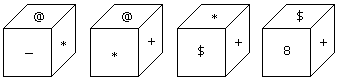Solution: The symbols of the adjacent faces to the face with symbol * are @, -, + and \$. Hence the required symbol is 8.
QUESTION: 2

### Two positions of dice are shown below. How many points will appear on the opposite to the face containing 5 points?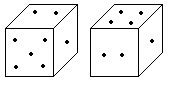Solution: In these two positions one of the common face having 1 point is in the same position. Therefore according to rule (2). There will be 4 points on the required face.
QUESTION: 3

### Which digit will appear on the face opposite to the face with number 4?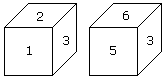Solution:

Here the common faces with number 3, are in same positions. Hence 6 is opposite to 2 and 5 is opposite to 1. Therefore 4 is opposite to 3.

QUESTION: 4

How many points will be on the face opposite to in face which contains 2 points?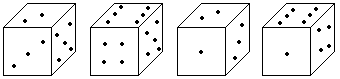Solution: In first two positions of dice one common face containing 5 is same. Therefore according to rule no. (3) the face opposite to the face which contains 2 point, will contains 6 points.
QUESTION: 5

Two positions of a dice are shown below. Which number will appear on the face opposite to the face with the number 5?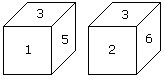Solution: According to the rule no. (3), common faces with number 3, are in same positions. Hence the number of the opposite face to face with number 5 will be 6.
QUESTION: 6

Which number is on the face opposite to 6?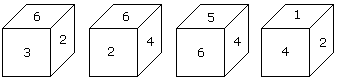Solution: As the numbers 2, 3, 4 and 5 are adjacent to 6. Hence the number on the face opposite to 6 is 1.
QUESTION: 7

Two positions of a dice are shown below. When number '1' is on the top. What number will be at the bottom?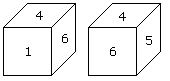Solution: According to the rule (2) when 'one' is at the top, then 5 will be at the bottom.
QUESTION: 8

Two positions of a cube with its surfaces numbered are shown below. When the surface 4 touch the bottom, what surface will be on the top?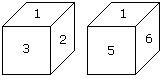Solution: In these 2 positions one common face with number 1 is in the same position. Hence according to the rule number (3), 2 is opposite 6 and 3 is opposite to 5. Therefore opposite to 4 is 1.
QUESTION: 9

Here two positions of dice are shown. If there are two dots in the bottom, then how many dots will be on the top?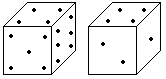Solution:

Here the common faces with 4 dots are in same positions.

Hence 2 will be opposite to 5.

QUESTION: 10

Two positions of dice are shown below. How many points will be on the top when 2 points are at the bottom?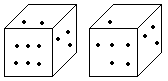Solution: In these 2 positions of a dice, one common face having points 3 is in the same position. Hence according to rule (3), there will be 4 points on the required face.
QUESTION: 11

Here 4 positions of a cube are shown. Which sign will be opposite to '+' ?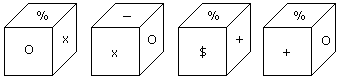Solution: From position I and III common face with % is in the same position. Hence according to rule (3) opposite is X.
QUESTION: 12

Two positions of a cubical block are shown. When 5 is at the top which number will be at bottom?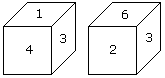Solution: In these 2 positions one common face with number 3, is in same position. Hence according to rule (3), 1 is opposite to 6 and 4 is opposite to 2. Therefore 5 is opposite to 3.
QUESTION: 13

From the four positions of a dice given below, find the color which is opposite to yellow ?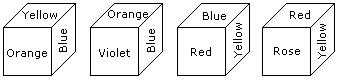Solution: The colours adjacent to yellow are orange, blue, red and rose. Hence violet will be opposite to yellow.
QUESTION: 14

When the digit 5 is on the bottom then which number will be on its upper surface?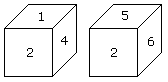Solution: According to the rule no. (3), common faces with number 2 are in same positions. Hence when the digit 5 is on the bottom then 1 will on the upper surface.
QUESTION: 15

How many points will be on the face opposite to the face which contains 3 points?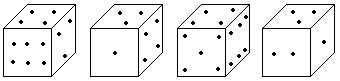Solution: The adjacent faces to the face which 3 points have 2, 1, 4 and 6 points. Hence on the face which is opposite to the face which contains 3 points, there will be 5 points.